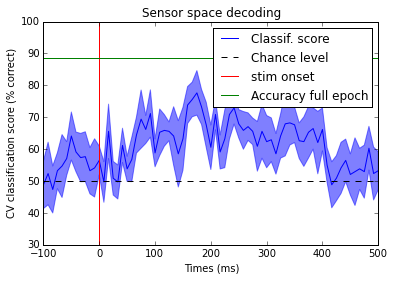# Mining brain waves with MNE and scikit-learn

## ... brain reading with MNE and scikit-learn

Author : Alexandre Gramfort alexandre.gramfort@telecom-paristech.fr

In :
# add plot inline in the page
%matplotlib inline
import matplotlib.pyplot as plt


In :
import mne


We set the log-level to 'WARNING' so the output is less verbose

In :
mne.set_log_level('WARNING')


## Access raw data¶

Now we import the dataset. If you don't already have it, it will be downloaded automatically (but be patient approx. 2GB)

In :
from mne.datasets import spm_face
data_path = spm_face.data_path()
raw_fname = data_path + '/MEG/spm/SPM_CTF_MEG_example_faces1_3D_raw.fif'


In :
raw = mne.io.Raw(raw_fname, preload=True)
print raw

<Raw  |  n_channels x n_times : 340 x 324474>


Band pass the data between 1Hz and 45Hz

In :
raw.filter(1, 45)

In :
%matplotlib osx
fig = raw.plot()


First extract events:

In :
%matplotlib inline
events = mne.find_events(raw, stim_channel='UPPT001', verbose=True)

172 events found
Events id: [1 2 3]


In :
mne.viz.plot_events(events, raw.info['sfreq'], raw.first_samp);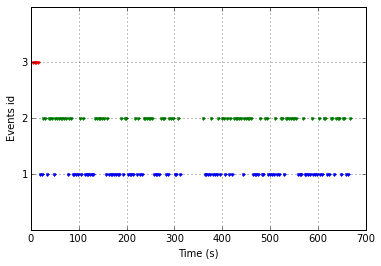## From raw to epochs

Define epochs parameters:

In :
event_id = {"faces": 1, "scrambled": 2}
tmin, tmax = -0.1, 0.5

# Set up pick list
picks = mne.pick_types(raw.info, meg=True, stim=True, eog=True,

decim = 4  # decimate to make the example faster to run
epochs = mne.Epochs(raw, events, event_id, tmin, tmax, proj=True,
reject=dict(mag=1.5e-12), decim=decim)

print epochs

<Epochs  |  n_events : 166 (all good), tmin : -0.1 (s), tmax : 0.5 (s), baseline : None,
'faces': 83, 'scrambled': 83>


Look at the ERF and contrast between left and rigth response

In :
evoked_faces = epochs['faces'].average()
evoked_scrambled = epochs['scrambled'].average()
evoked_contrast = evoked_faces - evoked_scrambled

In :
ylim = dict(mag=[-400., 400.])
fig = evoked_faces.plot(ylim=ylim)
fig = evoked_scrambled.plot(ylim=ylim)
fig = evoked_contrast.plot(ylim=ylim)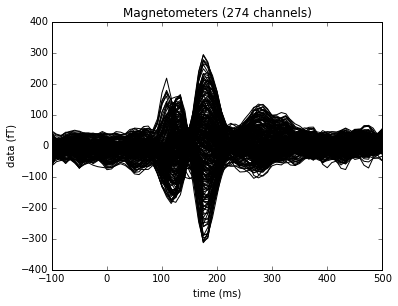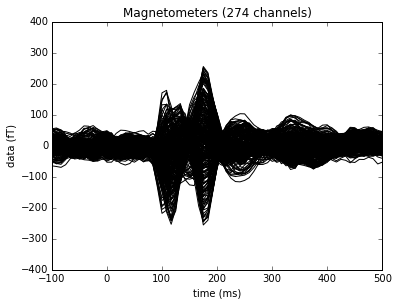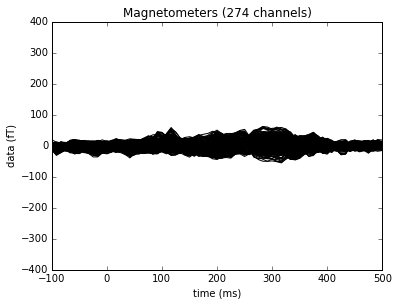Plot some topographies

In :
import numpy as np
times = np.linspace(-0.1, 0.3, 10)
fig = evoked_faces.plot_topomap(times=times, ch_type='mag', contours=0)
fig = evoked_scrambled.plot_topomap(times=times, ch_type='mag', contours=0)
fig = evoked_contrast.plot_topomap(times=times, ch_type='mag', contours=0)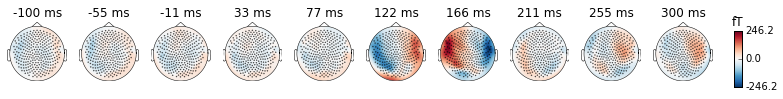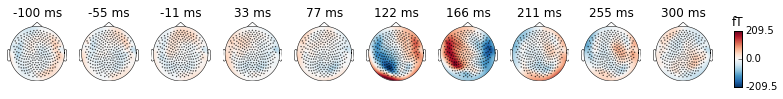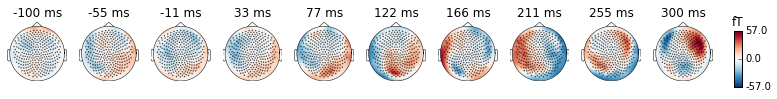## Now let's see if we can classify single trials with an SVM

To have a chance at 50% accuracy equalize epoch count in each condition

In :
epochs_list = [epochs[k] for k in event_id]
mne.epochs.equalize_epoch_counts(epochs_list)


### Format the data for scikit-learn

A classifier takes as input an x and return y (0 or 1). Here x will be the data at one or all time point(s) on all MEG sensors.

We work with all sensors jointly and try to find a discriminative pattern between 2 conditions to predict the class.

In :
n_times = len(epochs.times)

# Take only the data channels (here the gradiometers)

# Make arrays X and y such that :
# X is 3d with X.shape is the total number of epochs to classify
# y is filled with integers coding for the class to predict
# We must have X.shape equal to y.shape

X = [e.get_data()[:, data_picks, :] for e in epochs_list]
y = [k * np.ones(len(this_X)) for k, this_X in enumerate(X)]
X = np.concatenate(X)
y = np.concatenate(y)

In :
print X.shape, y.shape
print y

(166, 274, 73) (166,)
[ 0.  0.  0.  0.  0.  0.  0.  0.  0.  0.  0.  0.  0.  0.  0.  0.  0.  0.
0.  0.  0.  0.  0.  0.  0.  0.  0.  0.  0.  0.  0.  0.  0.  0.  0.  0.
0.  0.  0.  0.  0.  0.  0.  0.  0.  0.  0.  0.  0.  0.  0.  0.  0.  0.
0.  0.  0.  0.  0.  0.  0.  0.  0.  0.  0.  0.  0.  0.  0.  0.  0.  0.
0.  0.  0.  0.  0.  0.  0.  0.  0.  0.  0.  1.  1.  1.  1.  1.  1.  1.
1.  1.  1.  1.  1.  1.  1.  1.  1.  1.  1.  1.  1.  1.  1.  1.  1.  1.
1.  1.  1.  1.  1.  1.  1.  1.  1.  1.  1.  1.  1.  1.  1.  1.  1.  1.
1.  1.  1.  1.  1.  1.  1.  1.  1.  1.  1.  1.  1.  1.  1.  1.  1.  1.
1.  1.  1.  1.  1.  1.  1.  1.  1.  1.  1.  1.  1.  1.  1.  1.  1.  1.
1.  1.  1.  1.]


Now let's use an SVM to classify our MEG data

In :
from sklearn.svm import SVC
from sklearn.cross_validation import cross_val_score, ShuffleSplit

# Define an SVM classifier (SVC) with a linear kernel
clf = SVC(C=1., kernel='linear')


Define a monte-carlo cross-validation generator (to reduce variance):

In :
cv = ShuffleSplit(len(X), 10, test_size=0.2, random_state=42)


The goal is going to be to learn on 80% of the epochs and evaluate on the remaining 20% of trials if we can predict accurately.

In :
X_2d = X.reshape(len(X), -1)
X_2d = X_2d / np.std(X_2d)
scores_full = cross_val_score(clf, X_2d, y, cv=cv, n_jobs=1)
print "Classification score: %s (std. %s)" % \
(np.mean(scores_full), np.std(scores_full))

Classification score: 0.885294117647 (std. 0.0482388807849)


It's also possible to run the same classifier at each time point to know when in time the conditions can be better classified:

In :
scores = np.empty(n_times)
std_scores = np.empty(n_times)

from scipy.stats import zscore

X = zscore(X, axis=-1)  # standardize features
for t, Xt in enumerate(X.T):  # Run cross-validation
scores_t = cross_val_score(clf, Xt.T, y, cv=cv, n_jobs=1)
scores[t] = scores_t.mean()
std_scores[t] = scores_t.std()


A bit of rescaling

In :
times = 1e3 * epochs.times # to have times in ms
scores *= 100  # make it percentage accuracy
std_scores *= 100


Now a bit of plotting

In :
plt.plot(times, scores, label="Classif. score")
plt.axhline(50., color='k', linestyle='--', label="Chance level")
plt.axvline(0., color='r', label='stim onset')
plt.axhline(100. * np.mean(scores_full), color='g', label='Accuracy full epoch')
plt.legend()
hyp_limits = (scores - std_scores, scores + std_scores)
plt.fill_between(times, hyp_limits, y2=hyp_limits, color='b', alpha=0.5)
plt.xlabel('Times (ms)')
plt.ylabel('CV classification score (% correct)')
plt.ylim([30., 100.])
plt.title('Sensor space decoding')

Out:
<matplotlib.text.Text at 0x157196f50>